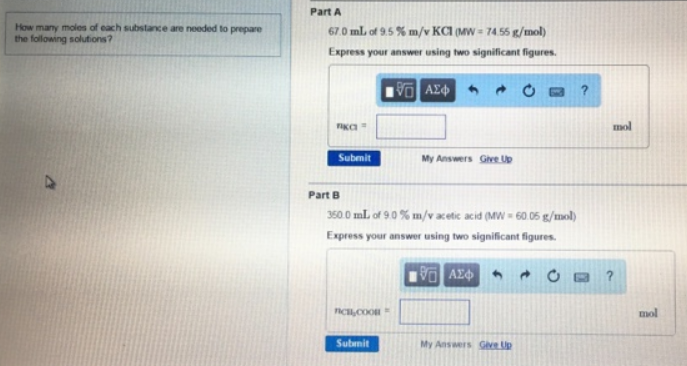# Problem: How many moles of each substance are needed to prepare the following solutions? Part A 67.0 mL of 9.5% m/v KCI (MW 74.55 g/mol)Express your answer using two significant figures. Part B 350.0 mL of 90%m/v acetic acid (Mw 60.06 g/mol) Express your answer using two significant figures.

###### FREE Expert Solution
82% (274 ratings)###### Problem Details

How many moles of each substance are needed to prepare the following solutions?

Part A 67.0 mL of 9.5% m/v KCI (MW 74.55 g/mol)

Part B 350.0 mL of 90%m/v acetic acid (Mw 60.06 g/mol)